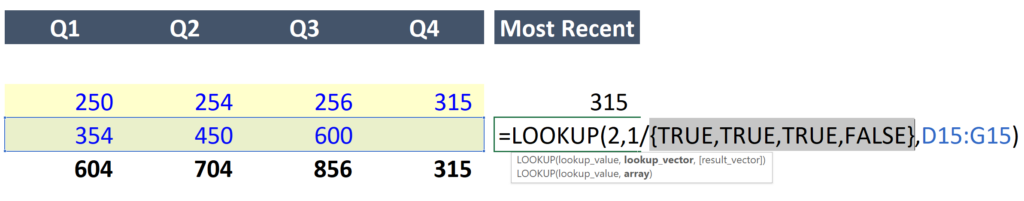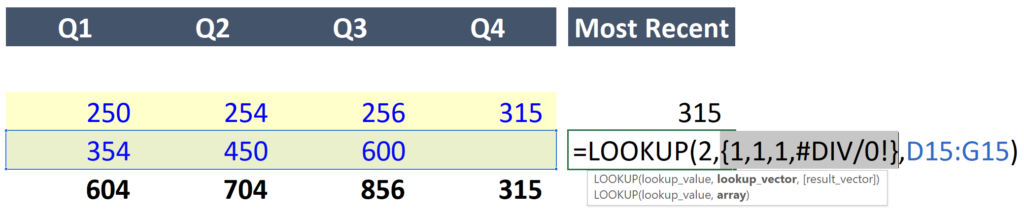Select Page

# Return the Last Non-Empty Value in a Row or Column in Excel

• Peter Lynch

In this post I will introduce a formula using the LOOKUP function that can pull the last non-empty cell value from a row or column in Excel. (Excel template available for download at the bottom of this post.)

To provide some context, assume you are updating your portfolio at the end of the year to see how your investments performed. Some of your investments report quickly but others lag. You require all of the most recent information in one column because it provides the most accurate total.

If you are here for the formula, I will save you the time: =LOOKUP(2,1/(cell_range<>””), cell_range). See image below:If you would like an explanation for how it works please see below. Before we explore how the LOOKUP function works, let’s first explore the operators in the formula.

#### What does <> mean in Excel?

The less than and greater than symbols in immediate succession (i.e., <>) mean “not equal to” in Excel.

#### What do quotation marks mean in Excel?

Quotation marks in Excel are used to denote the start and end of a text string. When quotation marks are combined with no characters in between, it states that the text string is empty.

So what we are saying here is that this cell range is NOT equal to an empty string. When you do that Excel returns an array. This array consists of True and False values per the image below.#### Combining it all with the LOOKUP function.

The result is an array of True or False values based on whether or not the cell range we selected contain a value.

Excel converts True and False values to 1 and 0 if you introduce a mathematical operation. So, we a value of one is included in the numerator to convert the array to ones and zeros.Then, with the LOOKUP function, we tell Excel to look for the value 2, which, obviously is never going to exist in this array, but excel will look through the array attempting to find this value and return the last value instead. Which in this case is the third position in the array.

The formula then uses the value returned from the lookup_vector, to return the desired value in the result_vector.